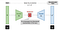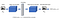# Image Anomaly Detection using Autoencoders

## Explore Deep Convolutional Autoencoders to identify Anomalies in Images.

This article is an experimental work to check if Deep Convolutional Autoencoders could be used for image anomaly detection on MNIST and Fashion MNIST.

## Autoencoder in a nutshell

Functionality: Autoencoders encode the input to identify important latent feature representation. It then decodes the latent features to reconstruct output values identical to the input values.

Objective: Autoencoder’s objective is to minimize reconstruction error between the input and output. This helps autoencoders to learn important features present in the data.

Architecture: Autoencoders consists of an Encoder network and a Decoder network. The encoder encodes the high dimension input into a lower-dimensional latent representation also referred to as the bottleneck layer. The decoder takes this lower-dimensional latent representation and reconstructs the original input.Source:https://lilianweng.github.io/lil-log/2018/08/12/from-autoencoder-to-beta-vae.html

Usage: Autoencoder are used for

• Dimensionality reduction
• Feature extractor
• Denoising images
• Image recognition and semantic segmentation
• Recommendation engines

Here we will use Tensorflow 2.3 and Fashion MNIST dataset.

To identify image anomalies, we will use the below architecture.Image by author

The encoder will compress the input data to its latent representation. The decoder will decompress the encoded latent representation to reconstruct the input data. The loss between the original input and the reconstructed input will be measured using the SSIM loss function. If the input belongs to images from the trained dataset then reconstruction loss will be smaller and if there is an anomaly then the reconstruction loss will be high.

SSIM is Structural Similarity Index Measure which is used to measure the similarity between two images. The SSIM value is between -1 and 1.

SSIM value is larger if two images are similar.

For image anomaly, we will use the SSIM loss function. For similar images, the SSIM loss function will be smaller and for anomalous images, the SSIM loss function will be larger.

Import required libraries

`import tensorflow as tfimport numpy as npimport matplotlib.pyplot as plt%matplotlib inline`

Load the Fashion MNIST train and test dataset, normalize and reshape to (28, 28,1)

`(x_train, _), (x_test, _) = fashion_mnist.load_data()x_train = x_train.astype('float32') / 255.x_test = x_test.astype('float32') / 255.x_train = np.reshape(x_train, (len(x_train), 28, 28, 1))x_test = np.reshape(x_test, (len(x_test), 28, 28, 1))`

Create the Encoder and Decoder

In the Encoder, you will compress the input data to its latent representation. The decoder will decompress the latent representation to recreate the input data.

`# Create the Encoder and Decoder#pass the gray scale input image of size(28,28,1)inputs = tf.keras.Input(shape=(28, 28, 1), name='input_layer')# Conv Block 1 -> BatchNorm->leaky Reluencoded = tf.keras.layers.Conv2D(32, kernel_size=3, strides= 1, padding='same', name='conv_1')(inputs)encoded = tf.keras.layers.BatchNormalization(name='batchnorm_1')(encoded)encoded = tf.keras.layers.LeakyReLU(name='leaky_relu_1')(encoded)# Conv Block 2 -> BatchNorm->leaky Reluencoded = tf.keras.layers.Conv2D(64, kernel_size=3, strides= 2, padding='same', name='conv_2')(encoded)encoded = tf.keras.layers.BatchNormalization(name='batchnorm_2')(encoded)encoded = tf.keras.layers.LeakyReLU(name='leaky_relu_2')(encoded)# Conv Block 3 -> BatchNorm->leaky Reluencoded = tf.keras.layers.Conv2D(64, kernel_size=3, strides=2, padding='same', name='conv_3')(encoded)encoded = tf.keras.layers.BatchNormalization(name='batchnorm_3')(encoded)encoded = tf.keras.layers.LeakyReLU(name='leaky_relu_3')(encoded)#Decoder# DeConv Block 1-> BatchNorm->leaky Reludecoded = tf.keras.layers.Conv2DTranspose(64, 3, strides= 1, padding='same',name='conv_transpose_1')(encoded)decoded = tf.keras.layers.BatchNormalization(name='batchnorm_4')(decoded)decoded = tf.keras.layers.LeakyReLU(name='leaky_relu_4')(decoded)# DeConv Block 2-> BatchNorm->leaky Reludecoded = tf.keras.layers.Conv2DTranspose(64, 3, strides= 2, padding='same', name='conv_transpose_2')(decoded)decoded = tf.keras.layers.BatchNormalization(name='batchnorm_5')(decoded)decoded = tf.keras.layers.LeakyReLU(name='leaky_relu_5')(decoded)# DeConv Block 3-> BatchNorm->leaky Reludecoded = tf.keras.layers.Conv2DTranspose(32, 3, 2, padding='same', name='conv_transpose_3')(decoded)decoded = tf.keras.layers.BatchNormalization(name='batchnorm_6')(decoded)decoded = tf.keras.layers.LeakyReLU(name='leaky_relu_6')(decoded)# outputoutputs = tf.keras.layers.Conv2DTranspose(1, 3, 1,padding='same', activation='sigmoid', name='conv_transpose_4')(decoded)`

The output layer uses a sigmoid activation function as it flattens the output to be in the range[0,1] which will be the same normalized input range for the grayscale input images.

Define SSIM loss function

`def SSIMLoss(y_true, y_pred):  return 1 - tf.reduce_mean(tf.image.ssim(y_true, y_pred,1.0))`

SSIM measures Structural Similarity between two images in terms of luminance, contrast, and structure. A value of 1 for SSIM indicates identical images. You want the SSIM loss function to be a minimum when training the autoencoder on good images.

Create the Autoencoder

`autoencoder = tf.keras.Model(inputs, outputs)optimizer = tf.keras.optimizers.Adam(lr = 0.0005)autoencoder.compile(optimizer=optimizer, loss=SSIMLoss)`

Train the Autoencoder

`hist=autoencoder.fit(x_train, x_train,                epochs=10,                batch_size=128,                shuffle=True,                validation_data=(x_test, x_test)                )`

Reconstruct the Fashion MNIST images for the test data and visualize

Pass the test dataset to the autoencoder and predict the reconstructed data. Visualize the original and the reconstructed images

`decoded_imgs = autoencoder.predict(x_test)n = 10plt.figure(figsize=(20, 4))for i in range(1, n + 1):    # Display original    ax = plt.subplot(2, n, i)    plt.imshow(x_test[i].reshape(28, 28))    plt.gray()    ax.get_xaxis().set_visible(False)    plt.title("Original")    ax.get_yaxis().set_visible(False)    # Display reconstruction    ax = plt.subplot(2, n, i + n)    plt.title("Reconstructed")    plt.imshow(decoded_imgs[i].reshape(28, 28))    plt.gray()    ax.get_xaxis().set_visible(False)    ax.get_yaxis().set_visible(False)plt.show()`

Finally, comparing the original data that the autoencoder was trained on, the reconstructed image of trained data, and prediction on the anomalous image using autoencoder.

`n = 6  # how many encoded and decoded images we will displaydecoded_imgs= autoencoder.predict(x_test)decoded_mnistimgs= autoencoder.predict(x_mnisttest)plt.figure(figsize=(20, 14), dpi=100)plt.subplots_adjust( wspace=0.1, hspace=0.07)plt_a=1for i in range(n):    # Original training dataset vs Original training    ax = plt.subplot(3, n, plt_a   )    plt.imshow(x_test[i].reshape(28,28))    ax.get_xaxis().set_visible(True)    ax.get_yaxis().set_visible(False)    value_a = SSIMLoss(x_test[i], x_test[i])    ax.set_title("Original Image")    label = 'SSIM Loss value: {:.3f}'    ax.set_xlabel(label.format(value_a) )        # Reconstructed good data  vs Original training data    ax = plt.subplot(3, n, plt_a + n )    plt.imshow(decoded_imgs[i].reshape(28,28))    ax.get_xaxis().set_visible(True)    ax.get_yaxis().set_visible(False)        value_a = SSIMLoss(decoded_imgs[i], x_test[i])    ax.set_title("Reconstructed Image")    label = 'SSIM Loss value: {:.3f}'    ax.set_xlabel(label.format(value_a) )        # Reconstructed anomalous data  vs Original training data    ax = plt.subplot(3, n, plt_a + 2*n)    plt.imshow(decoded_mnistimgs[i].reshape(28,28))    ax.get_xaxis().set_visible(True)    ax.get_yaxis().set_visible(False)    value = SSIMLoss(decoded_mnistimgs[i], decoded_imgs[i])    label = 'SSIM Loss value: {:.3f}'    ax.set_title("Anamolus Image " )    ax.set_xlabel(label.format(value) )    plt_a+=1plt.show()`

You can see from the above image that the SSIM loss is minimal for the reconstruction of the trained dataset however the SSIM loss is higher for the dataset the autoencoder was not trained on

## Conclusion:

Autoencoder works good for identifying anomaly with the grayscale MNIST and Fashion MNIST.

## References:

https://blog.keras.io/building-autoencoders-in-keras.html

## Analytics Vidhya

Analytics Vidhya is a community of Analytics and Data…

Written by

## Renu Khandelwal

Loves learning, sharing, and discovering myself. Passionate about Machine Learning and Deep Learning

## Analytics Vidhya

Analytics Vidhya is a community of Analytics and Data Science professionals. We are building the next-gen data science ecosystem https://www.analyticsvidhya.com

Written by

## Renu Khandelwal

Loves learning, sharing, and discovering myself. Passionate about Machine Learning and Deep Learning## Analytics Vidhya

Analytics Vidhya is a community of Analytics and Data Science professionals. We are building the next-gen data science ecosystem https://www.analyticsvidhya.com

## More From Medium

Medium is an open platform where 170 million readers come to find insightful and dynamic thinking. Here, expert and undiscovered voices alike dive into the heart of any topic and bring new ideas to the surface. Learn more

Follow the writers, publications, and topics that matter to you, and you’ll see them on your homepage and in your inbox. Explore

If you have a story to tell, knowledge to share, or a perspective to offer — welcome home. It’s easy and free to post your thinking on any topic. Write on Medium